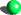# Math 254B lecture notes (Spring 2002)

The course is over, so the green ballindicating the current position of the course is no more. There are numerous errors in the notes that were corrected "on the fly" in class but have not been fixed in the notes. I subsequently compiled a corrected version of the lecture notes, which I recommend reading instead; however, for historical purposes I do plan to maintain the current page as it stands.

Explanation of references: the two main sources used in the course, which are cited throughout the notes without further comment, are Milne's notes on class field theory (available from Milne's web site) and Neukirch's book Algebraic Number Theory in its English translation (which was also the text for Math 254A, an introduction to algebraic number theory). To summarize, we follow Milne up through local class field theory, then recast local class field theory in an Artin-Tate-style abstract framework following Neukirch, then use that framework to develop global class field theory.

### Trailer: Abelian extensions of the rationals

The Kronecker-Weber theorem; a reciprocity law; reduction to the local case [TeX, DVI, PostScript]
Kummer theory; first glimpse of group cohomology [TeX, DVI, PostScript]
The local Kronecker-Weber theorem (revised 4 Feb) [TeX, DVI, PostScript]

### The statements of class field theory

Unramified abelian extensions and the Hilbert class field [TeX, DVI, PostScript]
Generalized ideal class groups; Artin reciprocity; ray class fields [TeX, DVI, PostScript]
The principal ideal ("capitulation") theorem [TeX, DVI, PostScript]
Zeta functions and L-functions; Dirichlet's theorem; the Chebotarev density theorem [TeX, DVI, PostScript]

### Cohomology of groups

Cohomology of finite groups I: abstract nonsense (exercise 2 corrected 22 Feb) [TeX, DVI, PostScript]
Cohomology of finite groups II: concrete descriptions, examples [TeX, DVI, PostScript]
Homology of finite groups, Tate groups, Herbrand quotient [TeX, DVI, PostScript]
Profinite groups, infinite Galois theory, (co)homology of profinite groups [TeX, DVI, PostScript]

### Local class field theory

Overview: the local reciprocity law, the local invariant map, interpretations [TeX, DVI, PostScript]
Cohomology of local fields: unramified, cyclic, general extensions [TeX, DVI, PostScript]
Putting it together: a theorem of Tate [TeX, DVI, PostScript]
Local CFT via "abstract class field theory" (a la Neukirch) [TeX, DVI, PostScript]
Addenda (corrects proof of local reciprocity law from previous handout) [TeX, DVI, PostScript]

The adele ring of a number field; the idele group; the idele class group [TeX, DVI, PostScript]
Extensions of adeles; trace and norm [TeX, DVI, PostScript]
The adelic reciprocity map and existence theorem; relationship with Artin reciprocity; what remains to be done [TeX, DVI, PostScript]

### The main results

The Herbrand quotient of the idele class group: the First Inequality [TeX, DVI, PostScript]
Cohomology of the idele class group: the Second Inequality [TeX, DVI, PostScript]
An "abstract" reciprocity law [TeX, DVI, PostScript]
The existence theorem [TeX, DVI, PostScript]
The connection with local reciprocity [TeX, DVI, PostScript]

### Coda

Parting thoughts [TeX, DVI, PostScript]
Kiran S. Kedlaya (kedlaya(at)math.berkeley.edu)